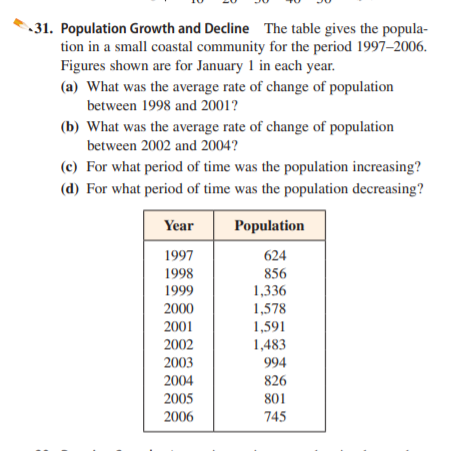# 31. Population Growth and Decline The table gives the popula- tion in a small coastal community for the period 1997–2006. Figures shown are for January 1 in each year. (a) What was the average rate of change of population between 1998 and 2001? (b) What was the average rate of change of population between 2002 and 2004? (c) For what period of time was the population increasing? (d) For what period of time was the population decreasing? Year Population 1997 1998 1999 624 856 1,336 1,578 1,591 1,483 2000 2001 2002 2003 994 2004 826 2005 801 2006 745

Question

. Population Growth and Decline The table gives the population in a small coastal community for the period 1997–2006.
Figures shown are for January 1 in each year.
(a) What was the average rate of change of population
between 1998 and 2001?
(b) What was the average rate of change of population
between 2002 and 2004?
(c) For what period of time was the population increasing?
(d) For what period of time was the population decreasing?help_outlineImage Transcriptionclose31. Population Growth and Decline The table gives the popula- tion in a small coastal community for the period 1997–2006. Figures shown are for January 1 in each year. (a) What was the average rate of change of population between 1998 and 2001? (b) What was the average rate of change of population between 2002 and 2004? (c) For what period of time was the population increasing? (d) For what period of time was the population decreasing? Year Population 1997 1998 1999 624 856 1,336 1,578 1,591 1,483 2000 2001 2002 2003 994 2004 826 2005 801 2006 745 fullscreen

### Want to see this answer and more?

Experts are waiting 24/7 to provide step-by-step solutions in as fast as 30 minutes!*

*Response times may vary by subject and question complexity. Median response time is 34 minutes for paid subscribers and may be longer for promotional offers.
Tagged in
Math
Algebra

### Other